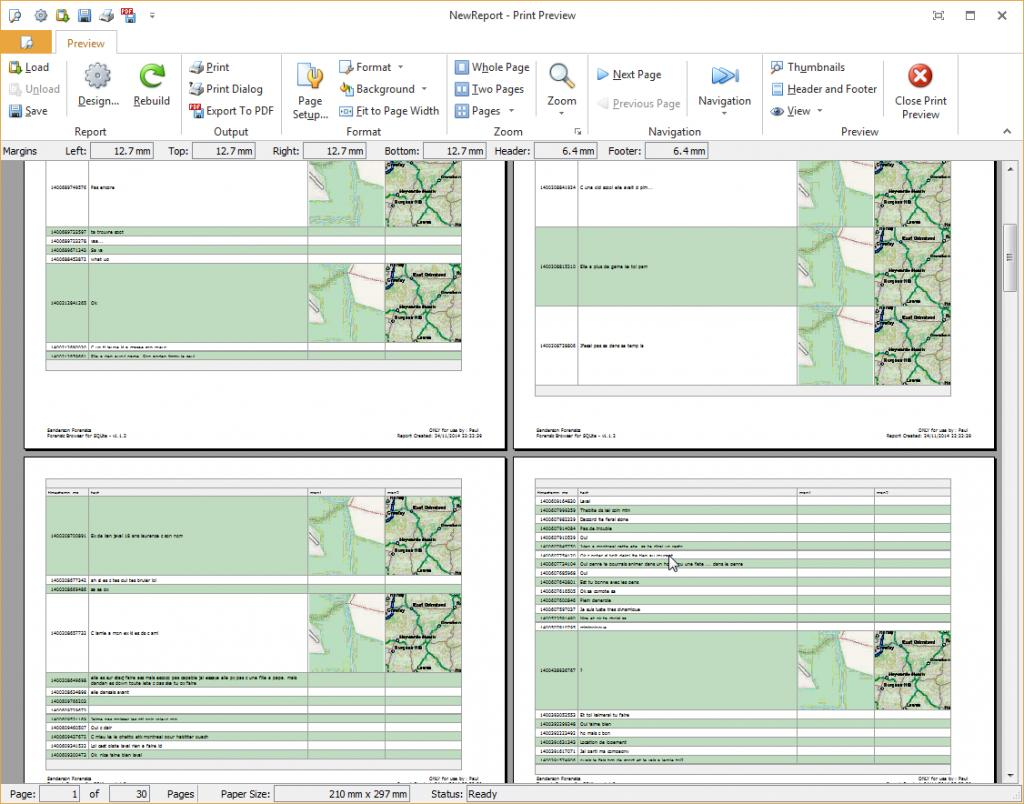I suspect that many of you have looked at Facebook SQLite databases and seen data in the messages table coordinates column of the form:

Code:

`{"longitude":-0.19690104212,"latitude":51.085777776363003,"accuracy":5}`

Since release 1.1.0 the Forensic Browser for SQLite has been able to display data stored in two columns, one for the latitude and one for the longitude as a map of the location.

In this article, I want to show how we can create a query using SQL that extracts the latitude and longitude (lat and long) from the single field above and create a temporary VIEW (a sort of temporary virtual table) that contains the lat and long. This VIEW can then be used to create a new permanent table (in the Forensic Browser case database) that holds three maps at different zoom levels that can be linked to the messages table.

First, we create a short query just to show the format of the text coordinates column from our database:

Code:

```SELECT messages.coordinates FROM messages WHERE messages.coordinates IS NOT NULL```

This query returns just the coordinates column for those rows where there are values in this column, as below: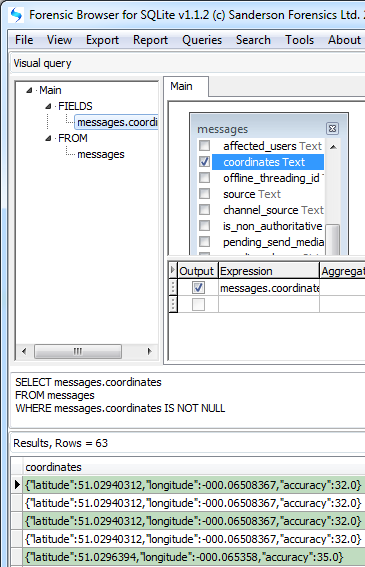Now we need to identify where the lat and long are in the text above. This is easy, they immediately follow the text ‘label’ before them i.e. the lon number starts 11 characters after the start of the word ‘longitude’ and the lat starts 10 characters after the word ‘latitude’.

So now we need to determine how long each number is, again this can be done by looking at each number again and seeing what follows it so the lon ends 2 characters before the start of the word ‘latitude’ and similar the lat ends two characters before the word ‘accuracy’. We need to extract these numbers. This diagram for the latitude calculation may make this clearer: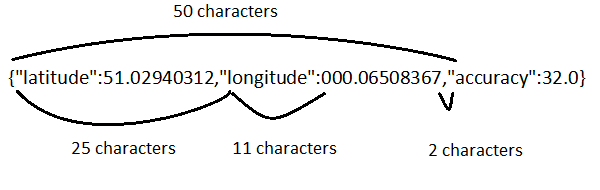The first digit of the longitude value starts 11 characters after the start of the word longitude which is 25 characters from the start of the text, i.e. 25 + 11 = 36. The end of the longitude starts 2 characters before the start of the word accuracy, i.e. 50 -2 = 48. Therefore the length of the longitude value is 48 – 36 = 12 characters

We can now utilize two of the SQLite core functions, first to identify the start offsets (characters) of the lat and long and second to extract the value.

The first function is Instr a function that finds the start of one string within another string (or field). So InStr(coordinates, ‘longitude’) will find the start of the word ‘longitude’. Note the single inverted comments around ‘longitude’ this instructs SQLite to consider longitude as a text string and not a field name.

The start offset of the longitude value is obtained with:

Code:

`InStr(messages.coordinates, 'longitude') + 11`

The end offset of the longitude value is obtained with:

Code:

`InStr(messages.coordinates, 'accuracy') - 2`

But we don’t want the end offset, we want the length of the longitude, we can get this by subtracting the start offset of the longitude value from the end offset, i.e.:

Code:

`(InStr(messages.coordinates, 'accuracy') - 2) – (InStr(messages.coordinates, 'longitude') + 11)`

We can then use the SubStr Function which as the name implies, extracts a substring from some text (or field), the second is InStr which finds the position of some supplied text from within some other text (or a field). So SubStr(coordinates, 36, 12) will extract the 12 characters of text starting at character 36, which we have just seen is the longitude field. We can substitute the expressions above into SubStr to get

Code:

`SubStr(messages.coordinates, InStr(messages.coordinates, 'longitude') + 11, (InStr(messages.coordinates, 'latitude') - 2) - (InStr(messages.coordinates, 'longitude') + 11))`

Now we need to devise an expression in the same way for the start of the latitude number and also the length of it. i.e.:

Code:

`SubStr(messages.coordinates, INSTR(messages.coordinates, 'latitude') + 10, (INSTR(messages.coordinates, 'longitude') - 2) - (INSTR(messages.coordinates, 'latitude') + 11))`

To add these to the SQL query we go to the fields editor and in the bottom blank expression box, we can type, or cut and paste the expression above.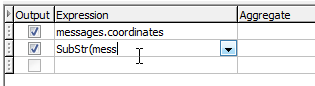When the expression has been entered we can change the alias column to read ‘Lon’.

In the screenshot below the Forensic Browser has fully qualified the field names by inserting the name of the table in front of the field name separated by a period (i.e. messages.).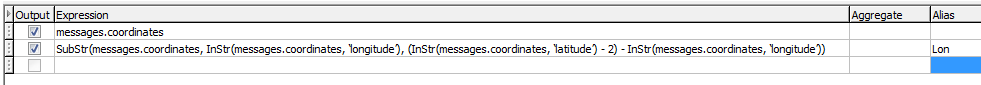The expression for latitude is derived in exactly the same way and should be entered in the next blank box:

Code:

```SubStr(coordinates, InStr(coordinates, ‘latitude') + 10, (InStr(coordinates, 'accuracy') - 2) - (InStr(coordinates, 'latitude') + 11))```

There is just one thing to add to the query before we can create our VIEW, that is to add a final field to the query that we can use to relate the lat and long back to the correct row in the messages table. This is almost invariably the primary key from the table from which we get our data, in the case of the messages table this is the msg_id field. Our final query becomes:

Code:

```SELECT messages.msg_id, messages.coordinates, SubStr(messages.coordinates, InStr(messages.coordinates, 'longitude') + 11, (InStr(messages.coordinates, 'latitude') - 2) - (InStr(messages.coordinates, 'longitude') + 11)) AS lon, SubStr(messages.coordinates, InStr(messages.coordinates, 'latitude') + 10, (InStr(messages.coordinates, 'accuracy') - 2) - (InStr(messages.coordinates, 'latitude') + 11)) AS lat FROM messages```

To create a VIEW on this table, execute the query and hit the F5 function key (or select “Create temp view on current query” from the Queries menu). When prompted enter a name for the VIEW, I have chosen “LatAndLongs”.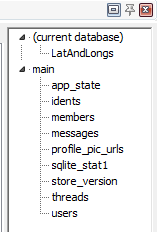Once the VIEW has been created it is then available to use as a virtual table and is displayed in the tables tree to the top right of the Forensic Reporter display, so queries can be run on it exactly as if it is a real table.

So the whole of the above complex query can now be replaced by:

Code:

`SELECT * from LatAndLongs`

Both of these SQL queries show exactly the same results, i.e.The next step is to turn these lat and long fields into maps. Provided the Forensic Browser for SQLite is connected to the internet you can do this by selecting “Create geolocated images” from the Tools menu.In the “Select Source Table” box type the name that you gave to the VIEW you have just created, in my case “LatAndlongs“, the Browser should auto-detect the two fields named lat and long (if you have used different names select them from the options provided). You also need to select (or enter) the ID field, i.e. the field that will link these, in this case it is msg_id as displayed below: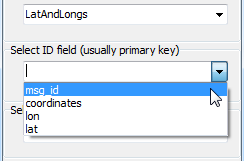Finally, you should either accept or type in a new table name for the destination data (the maps) – the default is Geodata1.

We can now run a query on Geodata1 to see the content of the table:

Code:

`SELECT * from geodata1`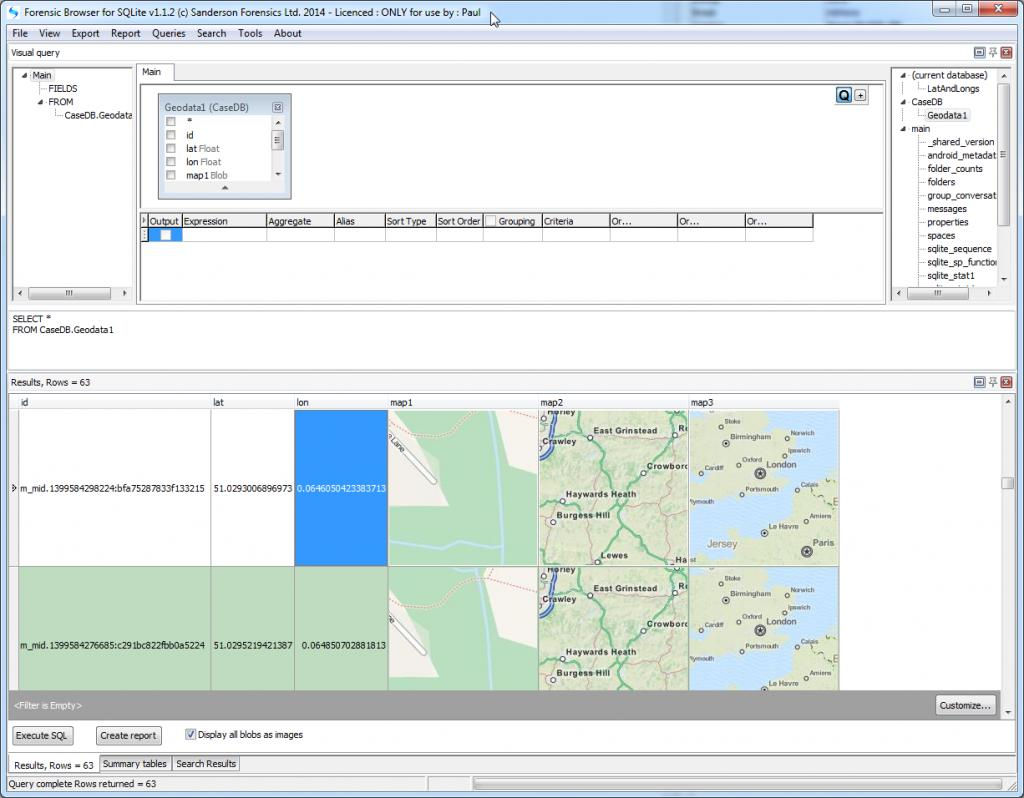All that remains to do now is create a query joining the messages table to the geodata1 table and select the columns (including the maps) that we want in our final report.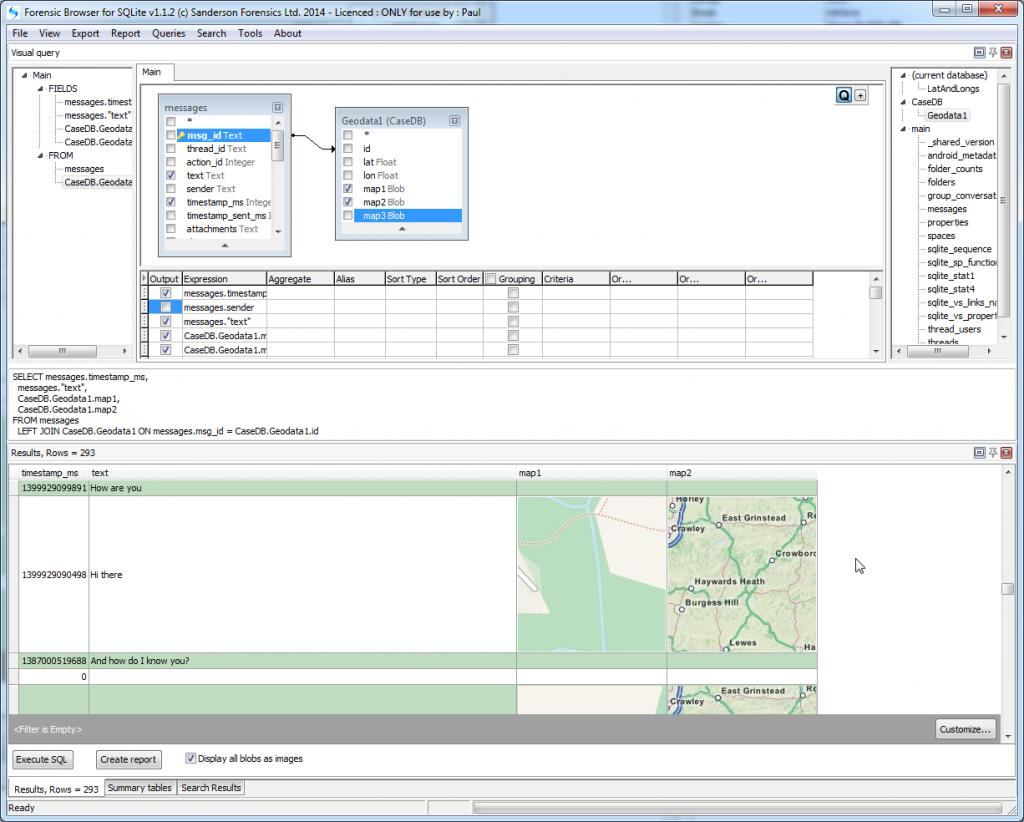And finally create the report: